NCERT Class 10 Maths Some Applications of Trigonometry

# NCERT Class 10 Maths Some Applications of Trigonometry

We have studied the basics of Trigonometry in the previous chapter and now let us study the applications of trigonometry to solve real-life problems. Here trigonometrical concepts are applied to determine heights and distances, angle of elevation, angle of depression and so on. and the relevant formulae studied in the previous chapter should be applied by visualizing the scenario stated in the problems. It has only one exercise where the focus of the problems is on the line of sight, angle of elevation and angle of depression. Thus, the height or length of an object or the distance between two distant objects can be determined with the help of trigonometric ratios.

## Chapter 9 Ex.9.1 Question 1

A circus artist is climbing a $$20\,\rm{m}$$ long rope, which is tightly stretched and tied from the top of a vertical pole to the ground. Find the height of the pole, if the angle made by the rope with the ground level is $$30^\circ$$ see Figure.

### Solution

What is Known?

(i) Length of rope $$=$$ $$20\,\rm{m}$$

(ii) Angle of rope with ground $$=$$ $$30^\circ$$$$=$$ $$\angle ACB$$

What is Unknown?

Height of pole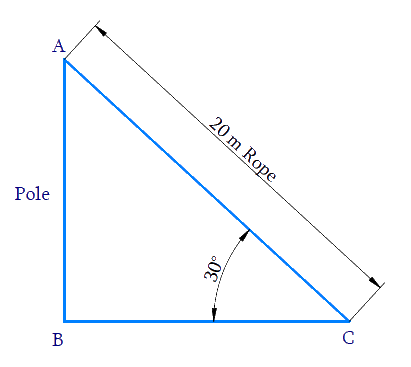Reasoning:

$$AB =$$ Height of the Pole.

$$BC =$$ Distance between the point on the ground and the pole.

$$AC =$$ Length of the Rope (Hypotenuse).

We need to find the height of the pole $$AB,$$ from the angle $$C$$ and the length of the rope $$AC.$$ Therefore, Trigonometric ratio involving all the three measures is $${\rm{sin}}\, C.$$

In $$\Delta ABC$$,

\begin{align} {AB} &=\frac{\text { opposite }}{\text { hypotenuse }} \\ {\rm{sin}}\,C &=\frac{{AB}}{{AC}} \\ {\rm{sin}}\, {C} &=\frac{{AB}}{20} \\ \frac{1}{2} &=\frac{{AB}}{20} \\ {AB}&=\frac{1}{\not{2}} \times \not\!\!{20}^{10}\ \\ {AB} &=10 \rm {m} \end{align}

Height of pole $$AB = 10\rm\,m.$$

## Chapter 9 Ex.9.1 Question 2

A tree breaks due to storm and the broken part bends so that the top of the tree touches the ground making an angle $$30^{\circ}$$ with it. The distance between the foot of the tree to the point where the top touches the ground is $$8\,\rm{m}$$. Find the height of the tree.

### Solution

#### What is Known?

(i) Broken part of the tree bends and touching the ground making an angle of $$30^{\circ}$$ with the ground.

(ii) Distance between foot of the tree to the top of the tree is $$8\,\rm{m.}$$

#### What is Unknown?

Height of the tree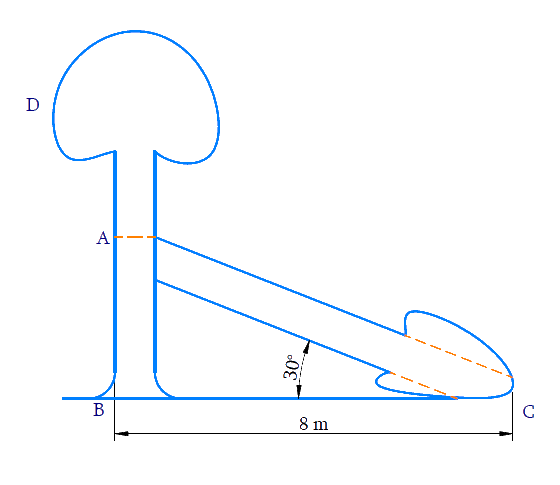#### Reasoning:

(i) Height of the tree $$= AB + AC.$$

(ii) Trigonometric ratio which involves $$AB, BC$$ and $$\angle C$$ is $$\tan \theta$$ , where $$AB$$ can be measured.

(iii) Trigonometric ratio which involves $$AB, AC$$ and $$\angle C$$ is $$\sin \theta$$ , where $$AC$$ can be measured.

(iv) Distance between the foot of the tree to the point where the top touches the ground $$= BC = 8\,\rm{ m}$$

#### Steps:

In $$\Delta ABC$$,

\begin{align}\tan C &=\frac{A B}{B C} \\ \tan 30^{\circ} &=\frac{A B}{8} \\ \frac{1}{\sqrt{3}} &=\frac{A B}{8} \\ A B &=\frac{8}{\sqrt{3}} \mathrm{m} \end{align}

\begin{align}\sin \,C{\rm{ }} &= {\rm{ }}\frac{{AB}}{{AC}}\\\sin 30^\circ &= \left( {\frac{{\frac{8}{{\sqrt 3 }}}}{{AC}}} \right)\\\frac{1}{2}{\rm{ }} &= {\rm{ }}\frac{8}{{\sqrt 3 }} \times \frac{1}{{AC}}\\AC{\rm{ }} &= {\rm{ }}\frac{8}{{\sqrt 3 }} \times 2\\AC{\rm{ }} &= {\rm{ }}\frac{{16}}{{\sqrt 3 }}\,\end{align}

\begin{align}\text{Height of tree}\, &= \rm{ }AB + AC\\&= \frac{8}{{\sqrt 3 }}+ \frac{{16}}{{\sqrt 3 }}\\&= \frac{{24}}{{\sqrt 3 }}\\&= \frac{{24}}{{\sqrt 3 }} \times \frac{{\sqrt 3 }}{{\sqrt 3 }}\\&= \frac{{24\sqrt 3 }}{3}\\&= 8\sqrt 3 \end{align}

Height of tree $$=8 \sqrt{3} \rm{m}$$

## Chapter 9 Ex.9.1 Question 3

A contractor plans to install two slides for the children to play in a park. For the children below the age of $$5$$ years, she prefers to have a slide whose top is at a height of $$1.5\,\rm{m}$$ and is inclined at an angle of $$30^\circ$$ to the ground, whereas for elder children she wants to have a steep slide at a height of $$3\,\rm{m}$$ and inclined at an angle of $$60^\circ$$ to the ground. What should be the length of the slide in each case?

### Solution

What is Known?

(i) For the children below the age of $$5$$ years.

Height of the slide $$=$$ $$1.5\,\rm{m}$$

Slide’s angle with the ground $$=$$ $$30^\circ$$

What is Unknown?

Length of the slide for children below the age of $$5$$ years and elder children.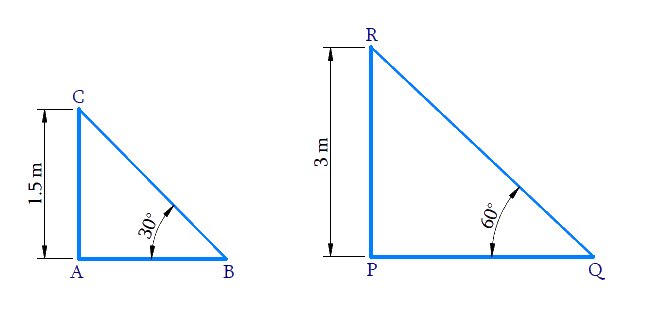(ii) For elder children.

Height of the slide $$= 3\,\rm{m}$$

Slide’s angle with the ground $$=$$$$60^\circ$$

Reasoning:

Let us consider the following conventions for the slide installed for children below $$5$$ years :

• The height of the slide as $$AC.$$
• Distance between the foot of the slide to the point where it touches the ground as $$AB.$$
• Length of the slide as $$BC.$$

Let us consider the following conventions for the slide installed for elder children:

• The height of the slide $$PR.$$
• Distance between the foot of the slide to the point where it touches the ground as $$PQ.$$
• Length of the slide as $$QR.$$

(i) Trigonometric ratio involving $$AC, BC$$ $$\angle B$$ and is $$\sin \theta$$

(ii) Trigonometric ratio involving $$PR, QR$$ and $$\angle Q$$ and is $$\sin \theta$$

Steps:

(i) In $$\Delta ABC$$,

\begin{align} \sin 30^{\circ} &=\frac{A C}{B C} \\ \frac{1}{2} &=\frac{1.5}{B C} \\ B C &=1.5 \times 2 \\ B C &=3 \mathrm{m}\end{align}

(ii) In $$\Delta \rm{PRQ}$$,

\begin{align}\sin \,Q &= \frac{{PR}}{{QR}}\\\sin \,60^\circ & = \frac{3}{{QR}}\\\frac{{\sqrt 3 }}{2}& = \frac{3}{{QR}}\\QR &= \frac{{3 \times 2}}{{\sqrt 3 }}\\&= \frac{6}{{\sqrt 3 }} \times \frac{{\sqrt 3 }}{{\sqrt 3 }}\\&= \frac{{6\sqrt 3 }}{3}\,\\&= 2\sqrt 3 \end{align}

Length of slide for children below $$5$$ years $$= 3\rm{m}.$$

Length of slide for elder children $$=$$ $$2 \sqrt3\,\rm{m}$$

## Chapter 9 Ex.9.1 Question 4

The angle of elevation of the top of a tower from a point on the ground, which is $$30\,\rm{m}$$ away from the foot of the tower, is $$30^\circ$$. Find the height of the tower.

### Solution

What is Known?

(i) Angle of elevation of the top of the tower from a point on ground is $$30^\circ$$

(ii) Distance between the foot of the tower to the point on the ground is $$30\,\rm{m.}$$

What is Unknown?

Height of the tower,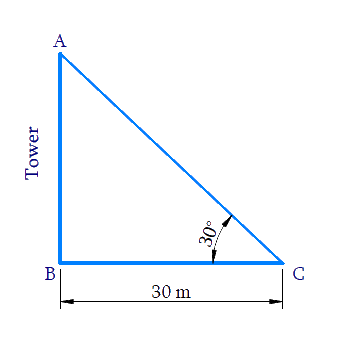Reasoning:

Let us consider the height of the tower as $$AB,$$ distance between the foot of tower to the point on ground as $$BC .$$

In $$\Delta ABC$$,

Trigonometric ratio involving $$AB, BC$$ and $$\angle C$$ is $$\tan \theta$$.

Steps:

In $$\Delta ABC$$,

\begin{align}\tan \,C &= \frac{{AB}}{{BC}}\\\tan 30^\circ &= \frac{{AB}}{{30}}\\\frac{1}{{\sqrt 3 }} &= \frac{{AB}}{{30}}\\AB &= \frac{{30}}{{\sqrt 3 }}\\&= \frac{{30}}{{\sqrt 3 }} \times \frac{{\sqrt 3 }}{{\sqrt 3 }}\\ &= \frac{{30\sqrt 3 }}{3}\\&= 10\sqrt 3 \end{align}

Height of tower $$AB$$ $$=10 \sqrt{3} \mathrm{m}$$

## Chapter 9 Ex.9.1 Question 5

A kite is flying at a height of $$60\,\rm{m}$$ above the ground. The string attached to the kite is temporarily tied to a point on the ground. The inclination of the string with the ground is $$60^\circ$$. Find the length of the string, assuming that there is no slack in the string.

### Solution

What is Known?

(i) Kite flying at a height $$= 60\,{\rm { m}}$$

(ii) Angle made by the string to the ground $$=60^\circ$$

What is Unknown?

Length of the string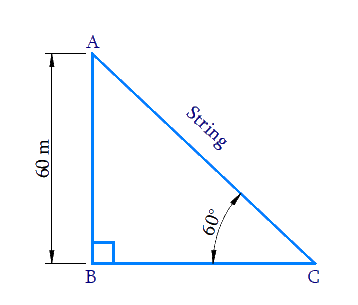Reasoning:

Let the height of the flying kite as $$AB$$, length of the string as $$AC$$ and the inclination of the string with the ground as $$\angle C$$

Trigonometric ratio involving $$AB, AC$$ and $$\angle C$$  is $$\sin \theta$$

Steps:

In $$\Delta ABC$$,

\begin{align}\sin \,C &= \frac{{AB}}{{AC}}\\\sin \,{60^0} &= \frac{{60}}{{AC}}\\\frac{{\sqrt 3 }}{2} &= \frac{{60}}{{AC}}\\AC &= \frac{{60 \times 2}}{{\sqrt 3 }}\\&= \frac{{120}}{{\sqrt 3 }} \times \frac{{\sqrt 3 }}{{\sqrt 3 }}\\&= \frac{{120\sqrt 3 }}{3}\\&= {\rm{40}}\sqrt {\rm{3}} \,\end{align}

Length of  the string $$AC =$$ $$40 \sqrt{3} \mathrm{m}$$

## Chapter 9 Ex.9.1 Question 6

A $$1.5\,\rm{m}$$ tall boy is standing at some distance from a $$30\,\rm{m}$$ tall building. The angle of elevation from his eyes to the top of the building increases from $$30^\circ$$ to $$60^\circ$$ as he walks towards the building. Find the distance he walked towards the building.

### Solution

What is Known?

(i) Height of the boy $$= 1.5\,{\rm{ m}}$$

(ii) Height of the building $$= 30\,{\rm {m}}$$

(iii) Angle of elevation from his eyes to the top of the building increases from $$30^\circ$$ to $$60^\circ$$ as he walks toward the building.

What is Unknown?

Distance, the boy walked towards the building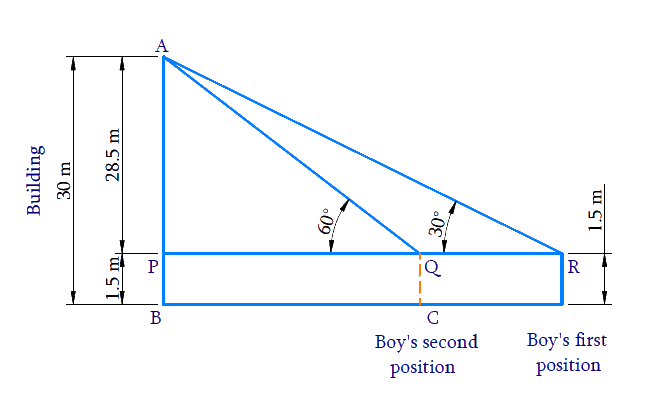Reasoning:

Trigonometric ratio involving ($$AP, PR$$ and $$\angle R$$) and ($$AP, PQ$$  and $$\angle Q$$) is $$tan \,\theta$$

[Refer thet  diagram to visualise $$AP, PR$$ and $$PQ$$]

Distance walked towards the building $$RQ$$ $$= PR – PQ$$

Steps:

In $$\Delta \mathrm{APR}$$

\begin{align} \tan R &=\frac{A P}{P R} \\ \tan 30^{\circ} &=\frac{28.5}{P R} \\ \frac{1}{\sqrt{3}} &=\frac{28.5}{P R} \\ P R &=28.5 \times \sqrt{3} \mathrm{m} \end{align}

In $$\Delta \mathrm{APQ}$$

\begin{align}{\tan \mathrm{Q}}&={\frac{A P}{P Q}} \\ {\tan 60^{\circ}}&={\frac{28.5}{P Q}}\\ \sqrt{3} &=\frac{28.5}{P Q} \\ P Q &=\frac{28.5}{\sqrt{3}} \mathrm{m} \end{align}

Therefore,

\begin{align}PR - PQ &= 28.5\sqrt 3 - \frac{{28.5}}{{\sqrt 3 }}\\ &= 28.5\left( {\sqrt 3 - \frac{1}{{\sqrt 3 }}} \right)\\&= 28.5\left( {\frac{{3 - 1}}{{\sqrt 3 }}} \right)\\&= 28.5\left( {\frac{2}{{\sqrt 3 }}} \right)\\&= \frac{{57}}{{\sqrt 3 }}\\&= \frac{{57}}{{\sqrt 3 }} \times \frac{{\sqrt 3 }}{{\sqrt 3 }}\\\rm&= \frac{{57\sqrt 3 }}{3}\\&= 19\sqrt 3 {\rm{m}}\end{align}

Distance the boy walked towards the building is $$19 \sqrt{3} \mathrm{m}$$.

## Chapter 9 Ex.9.1 Question 7

From a point on the ground, the angles of elevation of the bottom and the top of a transmission tower fixed at the top of a $$20\,\rm{m}$$ high building are $$45^\circ$$ and $$60^\circ$$ respectively. Find the height of the tower.

### Solution

What is Known?

(i) Angle of elevation from ground to bottom of the tower $$= 45^\circ$$

(ii) Angle of elevation from ground to top of the tower $$= 60^\circ$$

(iii) Height of the building $$= 20 \,\rm{m}$$

(iv) Tower is fixed at the top of the $$20\,\rm{m}$$ high building

What is Unknown?

Height of the tower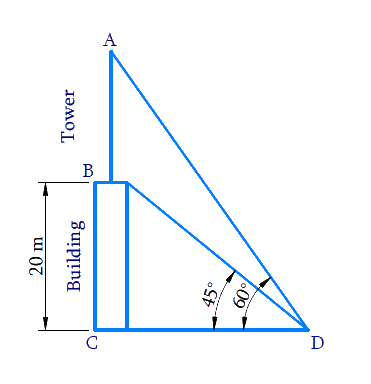Reasoning:

Let the height of the building is $$BC$$, height of the transmission tower which is fixed at the top of the building is $$AB$$. $$D$$ is the point on the ground from where the angles of elevation of the bottom $$B$$ and the top $$A$$ of the transmission tower $$AB$$ are $$45^\circ$$ and $$60^\circ$$ respectively.

The distance of the point of observation $$D$$ from the base of the building $$C$$ is $$CD$$

Combined height of the building and tower $$= AC = AB + BC$$

Trigonometric ratio involving sides $$AC,\, BC, \,CD$$ and $$∠D\, (45^\circ \rm{and}\, 60^\circ)$$ is $$\tan \theta$$

Steps:

In $$\Delta {BCD}$$

\begin{align}\tan 45^{\circ} &=\frac{B C}{C D} \\ 1 &=\frac{20}{C D} \\ C D &=20 \mathrm{m} \end{align}

In  $$\Delta {ACD}$$

\begin{align} \tan 60^{\circ} &=\frac{A C}{C D} \\ \sqrt{3} &=\frac{A C}{20} \\ A C &=20 \sqrt{3} \mathrm{m} \end{align}

Height of the tower,

$$AB = AC - BC$$

\begin{align}AB&=20\sqrt{3}\text m-20\text m \\ & =20\left( \sqrt{3}-1 \right)\rm m \end{align}

## Chapter 9 Ex.9.1 Question 8

A statue, $$1.6\,\rm{m}$$ tall, stands on the top of a pedestal, from a point on the ground. The angle of elevation of the top of the statue is $$60^\circ$$ and from the same point the angle of elevation of the top of the pedestal is $$45^\circ$$. Find the height of the pedestal.

### Solution

What is Known?

(i) Height of statue $$= 1.6 \,\rm{m}$$

(ii) Angle of elevation from ground to top of the statue $$= 60^\circ$$

(iii) Statue stands on the top of the pedestal.

(iv) Angle of elevation of the top of the pedestal (bottom of the statue) $$= 45^\circ$$

What is Unknown?

Height of the pedestal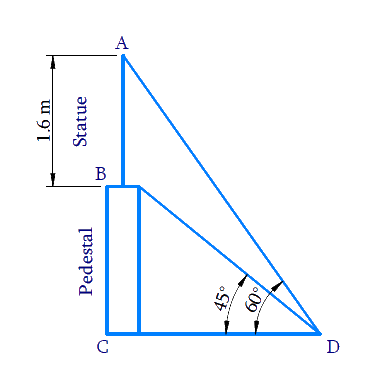Reasoning:

Let the height of the pedestal is $$BC$$, height of the statue, stands on the top of the pedestal, is $$AB$$. $$D$$ is the point on the ground from where the angles of elevation of the bottom $$B$$ and the top $$A$$ of the statue $$AB$$ are $$45^\circ$$ and $$60^\circ$$respectively.

The distance of the point of observation $$D$$ from the base of the pedestal $$C$$ is $$CD$$

Combined height of the pedestal and statue $$AC = AB + BC$$

Trigonometric ratio involving sides $$AC,\, BC, \,CD$$ and $$∠D$$ ($$45^\circ$$and $$60^\circ$$) is $$\tan \theta$$

Steps:

In $$\Delta BCD$$,

\begin{align}\tan {45^0} &= \frac{{BC}}{{CD}}\\1 &= \frac{{BC}}{{CD}}\\BC &= CD\quad\dots \left( {\rm{i}} \right)\end{align}

In  $$\Delta {ACD}$$

\begin{align}\tan {\rm{ }}{60^0} &= \frac{{AC}}{{CD}}\\\tan {\rm{ }}{60^0} &= \frac{{AB + BC}}{{CD}}\\\sqrt 3 &\!=\!\!\frac{{1.6 + BC}}{{BC}}\left[ {{\rm{from}}\left( {\rm{i}} \right)} \right]\\\sqrt 3 BC &= 1.6 + BC\\\sqrt 3 BC - BC &= 1.6\\BC\left( {\sqrt 3 - 1} \right) &= 1.6\\BC = \frac{{1.6}}{{\sqrt 3 - 1}} &\times \frac{{\sqrt 3 + 1}}{{\sqrt 3 + 1}}\\&= \frac{{1.6\left( {\sqrt 3 + 1} \right)}}{{3 - 1}}\\&= \frac{{1.6\left( {\sqrt 3 + 1} \right)}}{2}\\&= 0.8\left( {\sqrt 3 + 1} \right)\end{align}

Height of pedestal $$BC$$ $$=0.8(\sqrt{3}+1) \mathrm{m}$$

## Chapter 9 Ex.9.1 Question 9

The angle of elevation of the top of a building from the foot of the tower is $$30^\circ$$ and the angle of elevation of the top of the tower from the foot of the building is $$60^\circ.$$ If the tower is $$50\,\rm{m}$$ high, find the height of the building.

### Solution

What is Known?

(i) Angle of elevation of the top of a building from the foot of the tower  $$=30^\circ$$

(ii) Angle of elevation of the top of the tower from the foot of the building $$=60^\circ$$

(iii) Height of tower $$= 50\,{\rm{m}}$$

What is  Unknown?

Height of the buildingReasoning:

Let the height of the tower is $$AB$$ and the height of the building is $$CD$$. The angle of elevation of the top of the building $$D$$ from the foot of the tower $$B$$ is $$30^\circ$$ and the angle of elevation of the top of the tower $$A$$ from the foot of the building $$C$$ is $$60^\circ$$.

Distance between the foot of the tower and the building is $$BC$$.

Trigonometric ratio involving sides $$AB, \,CD, \,BC$$ and angles $$∠B$$ and $$∠C$$ is $$\tan \theta$$

Steps:

In  $$\Delta ABC$$

\begin{align}{\text{tan 6}}{{\rm{0}}^0} &= \frac{{AB}}{{BC}}\\\sqrt 3 &= \frac{{50}}{{BC}}\\BC &= \frac{{50}}{{\sqrt 3 }}\quad\dots\left( {\rm{i}} \right)\end{align}

In $$\Delta BCD$$,

\begin{align} \tan {{30}^{0}}&=\frac{CD}{BC} \\ \frac{1}{\sqrt{3}}&=\frac{CD}{BC} \\ \frac{1}{\sqrt{3}}&=\frac{CD}{\frac{50}{\sqrt{3}}}\qquad\left[ \text{from}\left( \text{i} \right) \right] \\ CD&=\frac{1}{\sqrt{3}}\times \frac{50}{\sqrt{3}} \\ CD&=\frac{50}{3} \\ CD&=16\frac{2}{3} \end{align}

Height of the building \begin{align}C D=16 \frac{2}{3} \mathrm{m}\end{align}

## Chapter 9 Ex.9.1 Question 10

Two poles of equal heights are standing opposite each other on either side of the road, which is $$80\,\rm{m}$$ wide. From a point between them on the road, the angles of elevation of the top of the poles are $$60^\circ$$ and $$30^\circ$$ respectively. Find the height of the poles and the distances of the point from the poles.

### Solution

What is Known?

(i) The poles of equal height.

(ii) Distance between poles $$= 80\,\rm{m}$$

(iii) From a point between the poles, the angle of elevation of the top of the poles are $$60^\circ$$ and $$30^\circ$$ respectively.

#### What is Unknown?

Height of the poles and the distances of the point from the poles.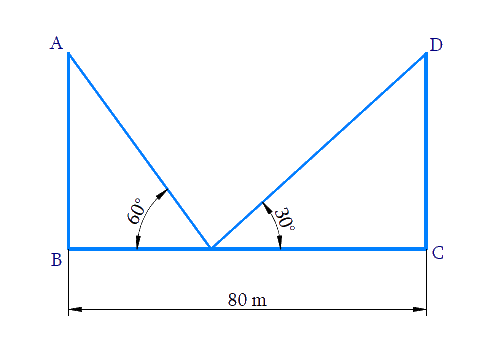Reasoning:

Let us consider the two poles of equal heights as $$AB$$ and $$DC$$ and the distance between the poles as $$BC$$. From a point $$O$$, between the poles on the road, the angle of elevation of the top of the poles $$AB$$ and $$CD$$ are $$60^\circ$$ and $$30^\circ$$ respectively.

Trigonometric ratio involving angles, distance between poles and heights of poles is $$\tan \theta$$

Steps:

Let the height of the poles be $$x$$

Therefore $$AB=DC=x$$

In $$\Delta AOB,$$

\begin{align}\text{tan 6}{{\text{0}}^{0}}&=\frac{AB}{BO} \\ \sqrt{3}&=\frac{x}{BO} \\ BO&=\frac{x}{\sqrt{3}}\quad\dots\left( \text{i} \right) \end{align}

In $$\Delta OCD,$$

\begin{align}& \tan \text{ }{{30}^{0}}=\frac{DC}{OC} \\& \frac{1}{\sqrt{3}}=\frac{x}{BC-BO} \\ & \frac{1}{\sqrt{3}}=\frac{x}{80-\frac{x}{\sqrt{3}}}\qquad\left[ \text{from }\left( \text{i} \right) \right] \\ & 80-\frac{x}{\sqrt{3}}=\sqrt{3}x \\ & \frac{x}{\sqrt{3}}+\sqrt{3}x=80 \\ & x\left( \frac{1}{\sqrt{3}}+\sqrt{3} \right)=80 \\ & x\left( \frac{1+3}{\sqrt{3}} \right)=80 \\ & x\left( \frac{4}{\sqrt{3}} \right)=80 \\ & x=\frac{80\sqrt{3}}{4} \\ & x=20\sqrt{3} \\ \end{align}

Height of the pole $$x = 20\sqrt 3 {\rm{ }}\,\rm m$$

Distance of point from $$O$$ the poles $$AB$$

\begin{align} BO&=\frac{x}{\sqrt{3}} \\ & =\frac{20\sqrt{3}}{\sqrt{3}} \\ & =20 \end{align}

Distance of point from $$O$$ the poles $$CD$$

\begin{align}OC&=BC-BO \\ & =80-20 \\ & =60 \end{align}

Height of the poles are  $$20\sqrt{3}\text{ }\rm{m}$$  and the distances of the point from the poles are $$20\rm{m}\text{ and }60\rm{m}$$ .

## Chapter 9 Ex.9.1 Question 11

A TV tower stands vertically on the bank of a canal. From a point on the other bank directly opposite the tower, the angle of elevation of the top of the tower is $$60^\circ$$. From another point $$20 \,\rm{m}$$ away from this point on the line joining this point to the foot of the tower, the angle of elevation of the top of the tower is $$30^\circ$$ see Figure. Find the height of the tower and the width of the canal.

### Solution

What is Known?

(i) The angle of elevation of the top of the tower from a point on the other bank directly opposite the tower is $$60^\circ$$

(ii) From another point $$20\,\rm{ m}$$ away from this point in (i) on the line joining this pointto the foot of the tower, the angle of elevation of the top of the tower is $$30^\circ$$

(iii)  $$CD =20\,\rm{ m}$$

What is Unknown?

Height of the tower $$=AB$$  and the width of canal $$=BC$$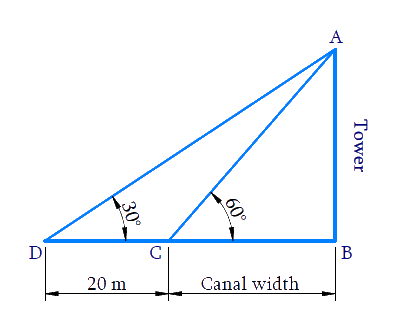Reasoning:

Trigonometric ratio involving $$CD,\, BC,$$ angles and height of tower $$AB$$ is $$tan\,\theta$$.

Steps:

Considering $$\Delta ABC,$$

\begin{align} \tan 60 ^ { \circ } & = \frac { A B } { B C } \\ \sqrt { 3 } & = \frac { A B } { B C } \\ A B & = \sqrt { 3 } B C \ldots . ( 1 ) \end{align}

Considering $$\Delta ABD,$$

\begin{align}\text{tan 3}{{\text{0}}^{0}}&=\frac{AB}{BD} \\ \text{tan 3}{{\text{0}}^{0}}&=\frac{AB}{CD+BC} \\ \frac{1}{\sqrt{3}}&\!\!=\!\!\frac{BC\sqrt{3}\,}{20+BC}{From}\,(1) \\ 20+BC&=BC\sqrt{3}\times \sqrt{3} \\ 20+BC&=3BC \\ 3BC-BC&=20 \\ 2BC&=20 \\ BC&=10\end{align}

Substituting$$BC = 10 \,\rm{m}$$ in Equation ($$1$$), we get

$$\text{AB = 10}\sqrt{\text{3}}\,\rm{m}$$

Height of the tower $$\text{AB = 10}\sqrt{\text{3}}$$

Width of the canal $$BC=10 \,\rm{m}$$

## Chapter 9 Ex.9.1 Question 12

From the top of a $$7 \,\rm{m}$$ high building, the angle of elevation of the top of a cable tower is $$60^\circ$$ and the angle of depression of its foot is $$45^\circ$$. Determine the height of the tower.

### Solution

What is Known?

(i) Height of building $$=7 \,\rm{m}$$

(ii) Angle of elevation of the top of a cable tower from building top $$=60^\circ$$

(iii) Angle of depression of the foot of the cable tower from building top $$= 45^\circ$$

What is Unknown?

Height of the tower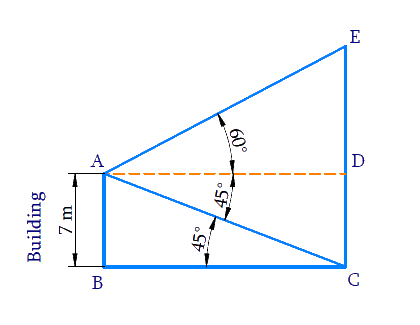Reasoning:

Let the height of the tower is $$CE$$ and the height of the building is $$AB$$. The angle of elevation of the top $$E$$ of the tower from the top $$A$$ of the building is $$60^\circ$$ and the angle of depression of the bottom $$C$$ of the tower from the top $$A$$of the building is $$45^\circ$$. Trigonometric ratio involving building height, tower height, angles and distances between them is $$\tan \theta$$

Steps:

Draw $$AD||BC$$.

Then,$$\angle DAC=\angle ACB={{45}^{0}}$$(alternate interior angles.)

In $$\Delta ABC$$,

\begin{align}\tan {{45}^{0}}&=\frac{AB}{BC} \\ 1&=\frac{7}{BC} \\ BC&=7 \end{align}

$$ABCD$$ is a rectangle,

Therefore, $$BC = AD = 7 \, \rm{m}$$ and $$AB = CD = 7 \, \rm{m}$$

In $$\Delta ADE$$,

\begin{align} { \tan 60 ^ \circ = \frac { E D } { A D } } \\ { \sqrt { 3 } = \frac { E D } { 7 } } \\ { E D = 7 \sqrt { 3 } } \end{align}

Height of tower

\begin{align}CE&=ED+CD \\ &=7\sqrt{3}+7 \\ &=7(\sqrt{3}+1) \end{align}

Height of the tower $$= 7 ( 1 + \sqrt { 3 } ) \,\rm{m}$$

## Chapter 9 Ex.9.1 Question 13

As observed from the top of a $$75\,\rm{m}$$ high lighthouse from the sea-level, the angles of depression of two ships are $$30^\circ$$ and $$45^\circ.$$ If one ship is exactly behind the other on the same side of the lighthouse, find the distance between the two ships.

### Solution

What is Known?

(i) Height of the lighthouse $$=75\,\rm{m}$$

(ii) Angles of depression of two ships from the top of the lighthouse are $$30^\circ$$ and $$45^\circ.$$

What is Unknown?

Distance between the two ships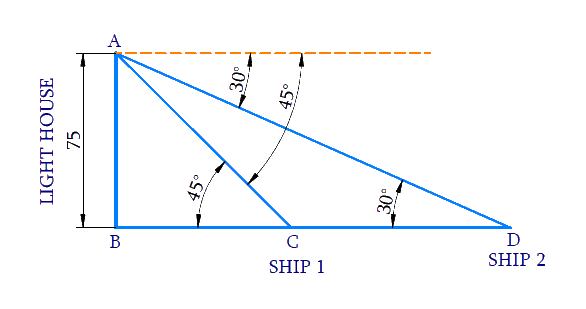Reasoning:

Let the height of the lighthouse from the sea-level is $$AB$$ and the ships are $$C$$ and $$D$$. The angles of depression of the ships $$C$$ and $$D$$ from the top $$A$$ of the lighthouse, are $$45^\circ$$ and $$60^\circ$$ respectively.

Trigonometric ratio involving $$AB, BC, BD$$ and angles is tan$$\theta$$.

Distance between the ships, $$CD = BD − BC$$

Steps:

In $$\Delta ABC$$,

\begin{align} \tan {{45}^{0}}&=\frac{AB}{BC} \\ 1&=\frac{75}{BC} \\ BC&=75\, \end{align}

In $$\Delta ABD$$,

\begin{align} \tan {{30}^{0}}&=\frac{AB}{BD} \\ \frac{1}{\sqrt{3}}&=\frac{75}{BD} \\ BD&=75\sqrt{3} \end{align}

Distance between two ships

$$CD=BD-BC$$

\begin{align}CD&=75\sqrt{3}-75 \\ &=75\left( \sqrt{3}-1 \right) \end{align}

Distance between two ships $$C D = 75 ( \sqrt { 3 } - 1 ) \, \rm{m}$$

## Chapter 9 Ex.9.1 Question 14

A $$1.2 \,\rm{m}$$ tall girl spots a balloon moving with the wind in a horizontal line at a height of $$88.2\,\rm{m}$$  from the ground. The angle of elevation of the balloon from the eyes of the girl at any instant is $$60^\circ.$$ After some time, the angle of elevation reduces to $$30^\circ$$ see Figure. Find the distance travelled by the balloon during the interval.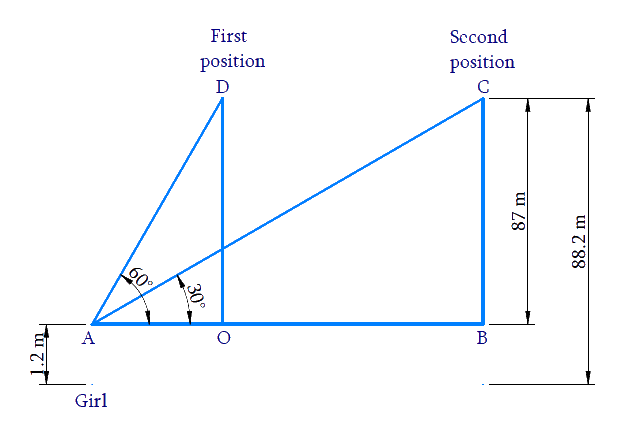### Solution

What is Known?

(i) Height of the girl $$=$$ $$1.2\,\rm{m}$$

(ii) Vertical height of balloon from ground $$=$$ $$88.2\,\rm{m}$$

(iii) Angle of elevation of the balloon from the eyes of the girl is reducing from $$60^\circ$$ to $$30^\circ$$ as the balloon moves along wind.

(iv) From the figure, $$OD = BC$$ can be calculated as

$$88.2\,\rm{m}$$ $$–$$ $$1.2\,\rm{m}$$ $$=$$ $$87\,\rm{m}\dots$$  Result (1)

What is Unknown?

Distance traveled by the balloon, $$OB$$

Reasoning:

Trigonometric ratio involving $$AB,\, BC,\, OD,\,OA$$ and angles is tan$$\theta$$. [Refer $$AB,\, BC,\, OA$$ and $$OD$$ from the figure.]

Distance travelled by the balloon $$OB = AB − OA$$

Steps:

From the figure, $$OD = BC$$, can be calculated as

$$88.2\rm {m}\, – 1.2 \rm {m} = 87 {m}$$---------------- ($$1$$)

In $$\Delta AOD,$$

\begin{align}\tan {{60}^{0}}&=\frac{OD}{OA} \\ \sqrt{3}&=\frac{87}{OA} \\ OA&=\frac{87}{\sqrt{3}} \\ &=\frac{87}{\sqrt{3}}\times \frac{\sqrt{3}}{\sqrt{3}} \\ &=\frac{87\times \sqrt{3}}{3} \\& =29\sqrt{3}\text{m} \end{align}

In $$\Delta ABC,$$

\begin{align}\tan {{30}^{0}}&=\frac{BC}{AB} \\ \frac{1}{\sqrt{3}}&=\frac{87}{AB} \\ AB&=87\sqrt{3} \end{align}

Distance traveled by the balloon $$OB = AB \,– OA$$

\begin{align}OB & =87\sqrt{3}-29\sqrt{3} \\ & =58\sqrt{3} \end{align}

Distance traveled by the balloon $$= 58 \sqrt { 3 } \, \,\rm{m}$$

## Chapter 9 Ex.9.1 Question 15

A straight highway leads to the foot of a tower. A man standing at the top of the tower observes a car at an angle of depression of $$30^\circ$$, which is approaching the foot of the tower with a uniform speed. Six seconds later, the angle of depression of the car is found to be $$60^\circ.$$ Find the time taken by the car to reach the foot of the tower from this point.

### Solution

What is Known?

(i) Angle of depression is $$30^\circ$$

(ii) $$6$$ seconds later angle of depression is $$60^\circ$$

What is Unknown?

Time taken by the car to reach the foot of the tower $$= CD$$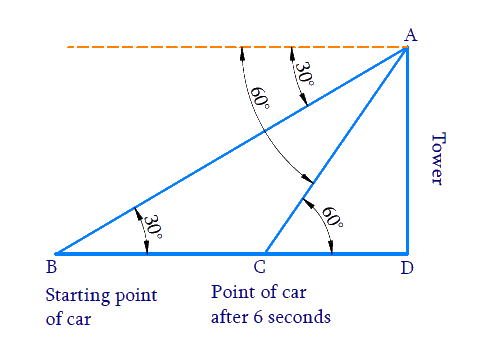Reasoning:

Let the height of the tower as $$AD$$ and the starting point of the car as $$B$$ and after $$6$$ seconds point of the car as $$C$$. The angles of depression of the car from the top $$A$$ of the tower at point $$B$$ and $$C$$ are $$30^\circ$$ and $$60^\circ$$ respectively.

Distance travelled by the car from the starting point towards the tower in $$6$$ seconds $$= BC$$

Distance travelled by the car after 6 seconds towards the tower $$= CD$$

Trigonometric ratio involving $$AD, \,BC, \,CD$$ and angles is $$tan\,\theta$$.

We know that,

$\text {Speed} = \frac { \text { Distance } } { \text { Time } }$

The speed of the car is calculated using the distance $$BC$$ and time $$= 6\,\rm seconds.$$ seconds. Using Speed and Distance $$CD,$$ time to reach foot can be calculated.

Steps:

In $$\Delta ABD$$,

\begin{align}\tan {{30}^{0}}&=\frac{AD}{BD} \\ \frac{1}{\sqrt{3}}&=\frac{AD}{BD} \\BD&=AD\sqrt{3}\,\,\,....(1) \end{align}

In $$\Delta ACD$$,

\begin{align} \tan {{60}^{0}}&=\frac{AD}{CD} \\ \sqrt{3}&=\frac{AD}{CD} \\ AD&=CD\sqrt{3}\,\,\,....(2)\text{ } \end{align}

From equation ($$1$$) and ($$2$$)

\begin{align}BD&=CD\sqrt{3}\times \sqrt{3} \\ BC+CD&=3CD\\&\left[ \because BD=BC+CD \right] \\ BC&=2CD\,\,\,\dots(3)\end{align}

Distance travelled by the car from the starting point towards the tower in $$6$$ seconds $$= BC$$ Speed of the car to cover distance $$BC$$ in $$6$$ seconds;

\begin{align}\text Speed&=\,\frac{\text{Distance}}{\text{Time}} \\ & =\,\frac{BC}{6\,} \\ &=\,\frac{2CD}{6}\,\qquad\left[ \text{from(3)} \right] \\ & =\,\frac{CD}{3}\, \end{align}

Speed of the car =\,\begin{align}\frac{CD}{3}\,\rm{m/s}\end{align}

Distance travelled by the car from point $$C$$, towards the tower $$= CD$$

Time to cover distance $$CD$$ at the speed of =\,\begin{align}\frac{CD}{3}\,\rm{m/s}\end{align}

\begin{align}\text Time &=\frac{\text{Distance}}{\text{Speed}} \\ & =\frac{CD}{\frac{CD}{3}}\, \\ & =CD\times \frac{3}{CD} \\ & =3 \end{align}

Time taken by the car to reach the foot of the tower from point $$C$$ is $$3$$ seconds.

## Chapter 9 Ex.9.1 Question 16

The angles of elevation of the top of a tower from two points at a distance of $$4\,\rm{m}$$  and $$9\,\rm{m}$$  from the base of the tower and in the same straight line with it are complementary. Prove that the height of the tower is $$6\,\rm{m.}$$

### Solution

What is Known?

Angle of elevation of the top of a tower from two points at a distance of $$4\,\rm{m}$$ and $$9\,\rm{m}$$ from the base of the tower are complimentary.

What is Unknown?

To prove height of the tower is $$6\,\rm{m.}$$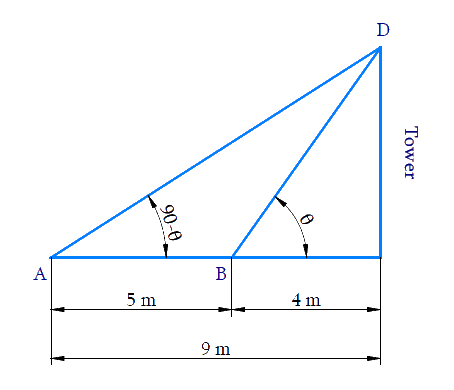Reasoning:

Let the height of the tower as $$CD$$. B is a point $$4\rm{m}$$ away from the base $$C$$ of the tower and $$A$$ is a point $$5\rm{m}$$ away from the point $$B$$ in the same straight line. The angles of elevation of the top $$D$$ of the tower from the points $$B$$ and $$A$$ are complementary.

Since the angles are complementary, if one angle is $$\theta$$ and the other is ($$90^\circ$$− $$\theta$$). Trigonometric ratio involving $$CD, \,BC,\, AC$$ and angles is $$tan\,\theta$$.

Using $$tan\,\theta$$ and tan( $$90^\circ$$ − $$\theta$$) $$=$$ $$cot\,\theta$$ ratios are equated to find the height of the tower.

Steps:

In $$\Delta BCD$$,

\begin{align}& \text{tan}\theta \,\text{= }\frac{CD}{BC} \\ & \text{tan}\theta \,\text{= }\frac{CD}{4}\quad\dots (1) \\ \end{align}

Here,

\begin{align}AC&=AB+BC \\ & =5+4 \\ & =9 \end{align}

In $$\Delta ACD$$,

\begin{align}{\rm{tan}}\left( {90 - \theta } \right)\,&= \frac{{CD}}{{AC}}\\\cot \theta &= \frac{{CD}}{9}\\&\left[ {\,{\rm{tan}}\left( {90 - \theta } \right)\, = \,\cot \theta } \right]\\\frac{1}{{{\rm{tan}}\theta }}\, &= \frac{{CD}}{9}\\&\left[ {\cot \theta = \frac{1}{{{\rm{tan}}\theta }}} \right]\\{\rm{tan}}\theta &= \frac{9}{{CD}}\quad \dots(2)\end{align}

From equation ($$1$$) and ($$2$$)

\begin{align}\frac{{CD}}{4} &= \frac{9}{{CD}}\\C{D^2} &= 36\\CD &= \pm 6\end{align}

Since, Height cannot be negative

Therefore, height of the tower is $$6\,\rm{m.}$$

Some Applications of Trigonometry | NCERT Solutions
Instant doubt clearing with Cuemath Advanced Math Program